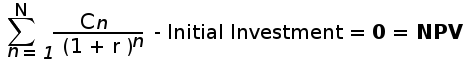# CAGR vs IRR :

CAGR (Compound annual growth rate) and IRR (Internal rate of return) are two important financial metric used for calculating investment return.

## Definition :

The compound annual growth rate or CAGR is the rate at which an investment grows over a certain period of time with the value compounding over that time.

The internal rate of return or IRR is defined in terms of NPV. IRR is the interest rate (discount rate) that makes the NPV (Net Present Value) of all cash flow equals to Zero.

## Calculation Formula :

The formula for CAGR is:

CAGR = ( EV / SV)1 / n - 1

where:

EV = Investment's ending value
SV = Investment's starting value
n = Number of investment periods (months, years, etc.)

The formula for calculating IRR is expressed in terms of NPV.Where in the above formula :

N = total number of periods

n = positive integer

C = cash flow

r = internal rate of return

NPV = net present value

## Comparison & Difference:

1. CAGR doesn't take into account the investment made periodically. It takes only the initial investment amount and final value for calculating the growth rate. Unlike CAGR, IRR takes the periodic cash-flow both positive and negative into consideration for calculation.

2. Calculation of CAGR is simple in comparison to IRR as it accounts the only initial and final value to calculate.

3. IRR is preferred over CAGR for evaluating complex or large projects which often have many positive and negative cash-flows. In such cases, IRR represents more accurate value.

4. In financial world, the IRR is more realistic than CAGR because it considers periodic cash-flows instead of one time lump-sum investment.

## Conclusion

As mentioned above, IRR gives clear picture for evaluating an investment or project. So, IRR is more preferred and accurate financial tool for evaluating return and risk of investment.

Read More : NPV vs IRR

You might like Our Financial Calculators:

Disclaimer:

The IRR Calculator provides "IRR" or "internal rate of return" without any warranty for it's accuracy. Any reliance by you on any information or advice will be at your own risk. Every decisions should be made after consultation with your financial advisor or professional.This website is not responsible for, and expressly disclaims all liability for, damages of any kind arising out of use, reference to, or reliance on any information contained within the site. By using this website you agree to those terms, if not then do not use this website.